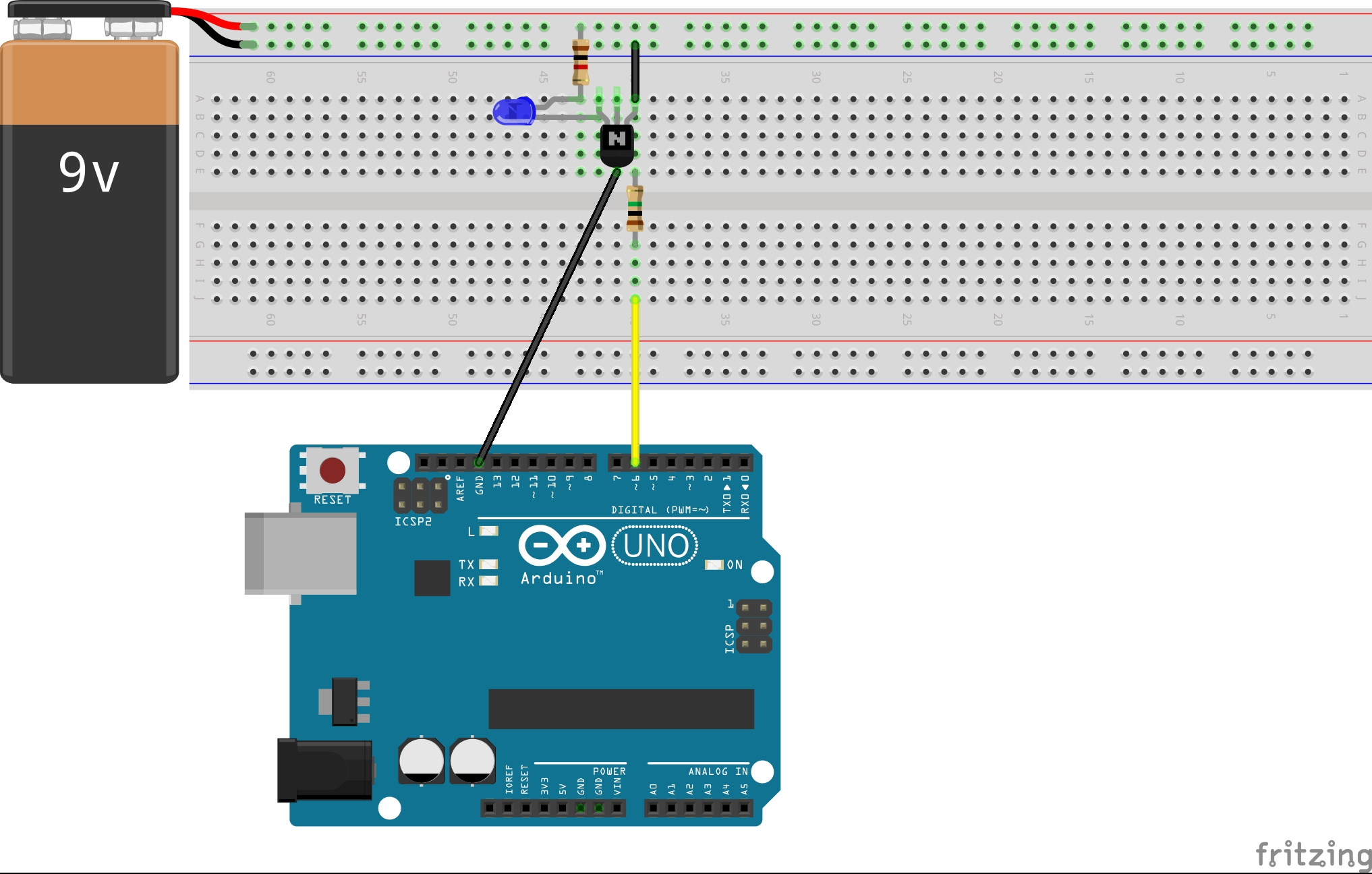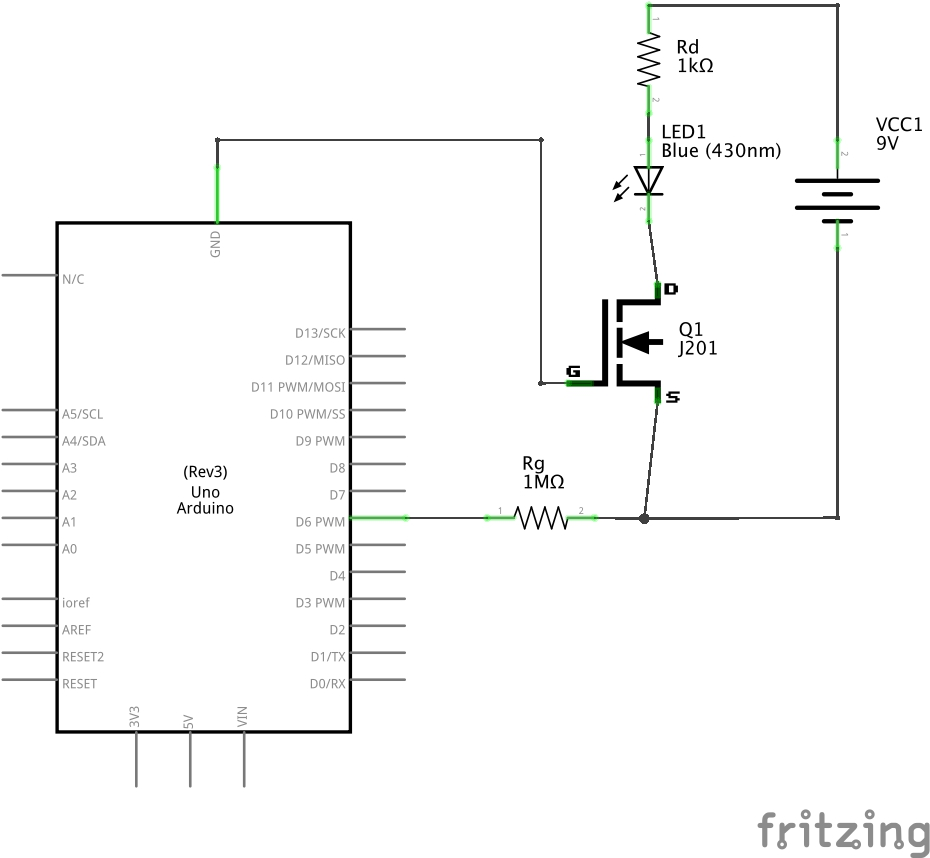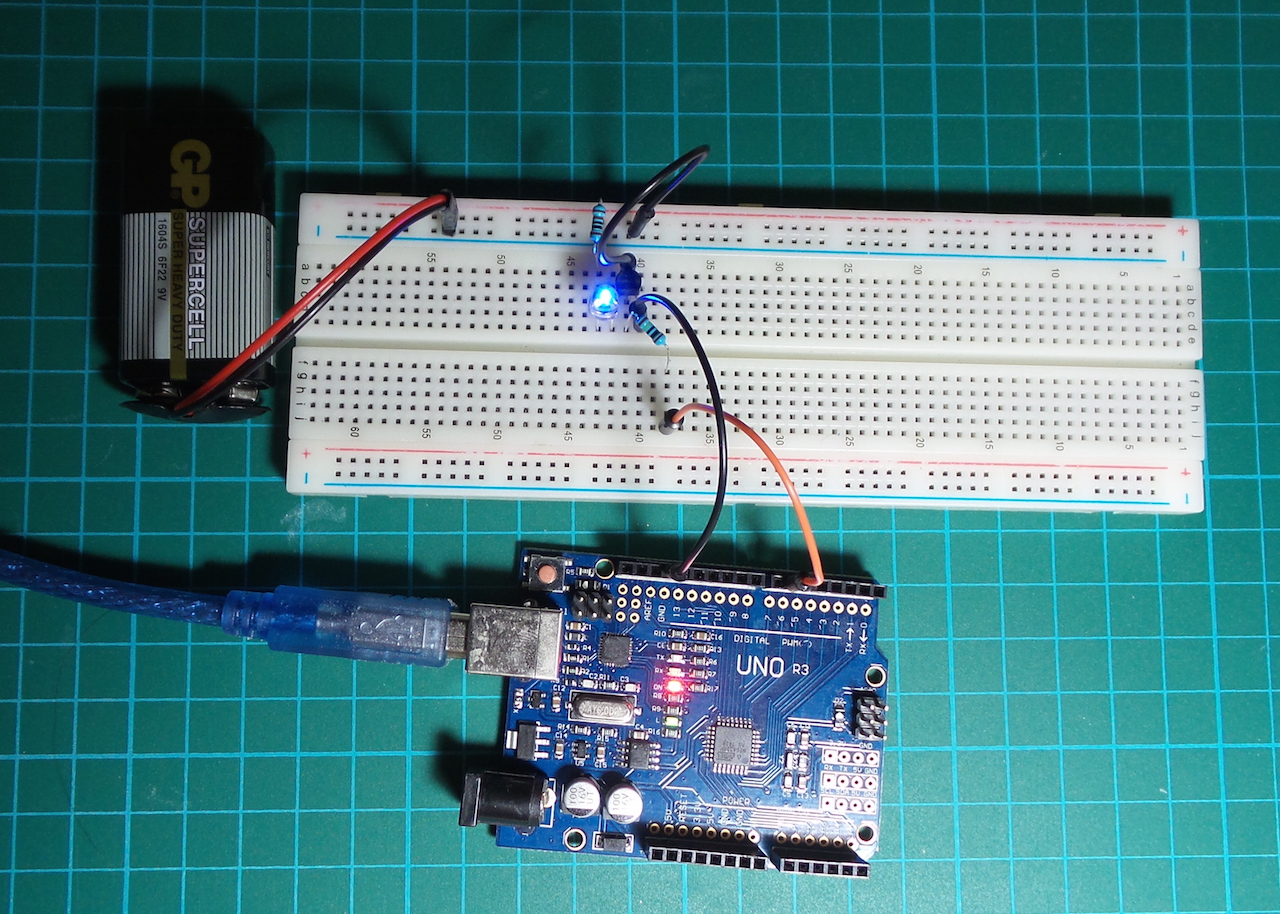# #116 SwitchNJFET

Switch an independent power source using an Arduino and n-channel JFET.

This is a simple switching circuit using an n-channel JFET. The JFET I’m using is the J201 which is intended for low-power applications with maximum ratings of 40V/50mA.

What makes n-channel JFETs interesting (and challenging?) is the need for a negative gate-source voltage (Vgs) to turn them “off”. Since drain voltage is higher than source voltage when on, this means the voltages are typically stacked: `drain > source >= gate`, and biasing the device often involves two power supplies.

In this circuit, I wanted to use the 5V Arduino to switch a 9V circuit. It turns out to be very easy, but caution is required to avoid inappropriate cross-connections of the circuit which may inadvertently subject the Arduino to excessive or reversed voltages.

The load circuit is a simple 9V loop with LED indicator and sufficient resistance to limit the current. The JFET source-drain loop is the switch in the circuit.

To bias the gate using a digital pin of the Arduino, we take the source of the JFET as the Arduino “positive”, and ground the Arduino at the gate. So the Arduino is able to apply from 0V to 5V the source with respect to gate. From the characteristic curves in the datasheet, we can see this is enough to effect a distinct on/off transition

• Vgs = 0V, the JFET is fully “on”
• Vgs = -5V, the JFET is fully “off”

### Rg - gate resistor

A 1MΩ resistor connects to the gate. In normal operation, this is redundant as the gate current is virtually zero (~1nA). i.e., yes the circuit still works fine with it removed.

It does seem to be good protection in case Vgs becomes reverse biased and significant current flow through the gate is possible.

### The Code

… doesn’t do anything special - just an amplified Blink! It uses a digital out pin to turn the JFET on and off.

## ConstructionNB: in the schematic below, the FET symbol is not quite correct (shows n-channel MOSFET instead of JFET)